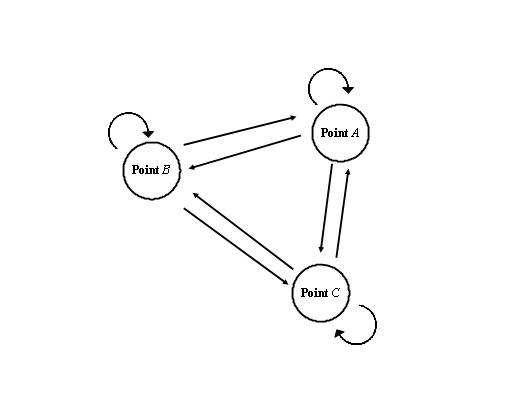# Many-worlds interpretation and the Born rule

2014/9/18
Published 2008/3/9

Many-worlds interpretation (MWI) has a problem we need to solve. The problem is that there is no method to derive the Born rule by MWI. On this page, I would like to explain the problem and introduce the ideas to derive the Born rule.## The Born rule

The observation probability is proportional to the absolute square of the wave function. This fact is called the Born rule. However, this rule is not derived theoretically. It is derived from the experimental facts.

Many people seem to be not interested in this problem. This may be because it is written as a basic principle in textbooks. However, I think that it is the important problem we need to solve.

## Many-worlds interpretation and the Born rule

Some people think that they can derive the Born rule by MWI. However, I do not think so.

American physicist Hugh Everett proposed many-worlds interpretation in 1957. He introduced a new concept measure in his paper. The measure is proportional to the absolute square of the wave function. He described that the measure behaves in the same way as probability.

He did not declare that the measure is proportional to the number of the worlds. However, I think that we should explain the probability using a concept of the number of the worlds if we adopt many-worlds interpretation.

The measure exists as a physical entity because the measure is proportional to the number of the worlds. On the other hand, the wave function exists as a physical entity because the wave function interferes.

I think that it is a key for the derivation of the Born rule to show the relation between the above two physical entities.

## Ideas for the derivation of the Born rule

We think the ideas to show the relation between the wave function and the measure. On the other hand, the measure is proportional to the absolute square of the wave function. Therefore, I would like to think the ideas that a quantity of a physical entity and the square of the quantity exist. The ideas are shown below.

1. The length of a spring and the energy of the spring.
2. The radius of a circle and the area of the circle.
3. The radius of a sphere and the surface area of the sphere.
4. The number of the points and the number of the path between each point.

A spring exists as a physical entity in the first idea. The absolute value of the wave function is the length of the spring. The number of the worlds is proportional to the energy of the spring.

A circle exists as a physical entity in the second idea. The absolute value of the wave function is the radius of the circle. The number of the worlds is proportional to the area of the circle.

A sphere exists as a physical entity in the third idea. The absolute value of the wave function is the radius of the sphere. The number of the worlds is proportional to the surface area of the sphere.

Points and paths exist as physical entities in the fourth idea. The absolute value of the wave function is the number of the points. The number of the worlds is the number of the paths. There are nine paths for three points in the following figure.I think that the fourth idea of the points and paths is convincing. I hope that this page make a chance to think about the Born rule in the many-worlds interpretation.

Related Articles: